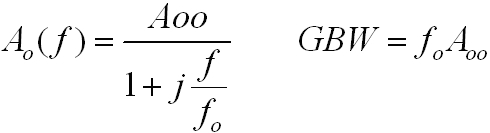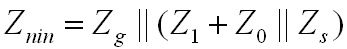# Inverting Amplifier with T-Network Analysis

Sep 2015The transfer function Av(f)=Vo/Vs for the circuit can be analyzed using standard node analysis with complex impedances. For this t-network configuration, it is slightly easier to transform the feedback network first to a Thevenin equivalent circuit from the op-amp output to the op-amp inverting input and proceed from that point. An ideal op-amp is assumed except for a finite GBW product. However, op-amp input resistance and capacitance can be included via Rg, Cg. The op-amp inputs are ideal and draw no current, and the op-amp output has zero impedance. The complex impedances Zi in the expression are parallel combinations of the corresponding Ri and Ci values except for Rs which is the series combination of Rs and Cs. In fact the expression is more general and Zi can correspond to any complex impedance between the terminals of the corresponding Ri/Ci component.

The voltage gain is:where the op-amp open-loop gain profile is:where the ideal voltage gain (with infinite GBW product for op-amp) is:The Noise Gain function (1/β(f)), which combined with Ao(f) ultimately limits the circuit bandwidth, unless the feedback network rolls the response off at a lower frequency, is:where Znin is the total impedance at the inverting input looking BACK at the circuit: